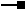# IEnvelope2 Interface

## Description

IEnvelope2 allows the minimum and maximum M and Z attributes of the Envelope to be queried and set simultaneously to avoid complications and inconsistencies of setting them individually.

## Members

Name DescriptionCenterAt Moves this envelope so it is centered at p.DefineFromPoints Defines the envelope to cover all the points.DefineFromWKSPoints Defines the envelope to cover all the points.Depth The depth of the envelope.Dimension The topological dimension of this geometry.Envelope Creates a copy of this geometry's envelope and returns it.Expand Moves the X and Y coordinates of the sides toward or away from each other.ExpandM Moves the measure of the sides toward or away from each other.ExpandZ Moves the Z attribute of the sides toward or away from each other.GeometryType The type of this geometry.GeoNormalize Shifts longitudes, if need be, into a continuous range of 360 degrees.GeoNormalizeFromLongitude Normalizes longitudes into a continuous range containing the longitude. This method is obsolete.Height The height of the envelope.Height The height of the envelope.Intersect Adjusts to include only the area also included by inEnvelope.IsEmpty Indicates whether this geometry contains any points.LowerLeft The lower left corner.LowerRight The lower right corner.MinMaxAttributes A reference to the attribute structures for this envelope.MMax The maximum measure value in the area of the envelope.MMin The minimum measure value in the area of the envelope.Offset Moves the sides x units horizontally and y units vertically.OffsetM Moves the sides m units.OffsetZ Moves the sides z units.Project Projects this geometry into a new spatial reference.PutCoords Constructs an envelope from the coordinate values of lower, left and upper, right corners.PutMCoords Sets the minimum and maximum M values simultaneously.PutWKSCoords Copies e's dimensions into this envelope.PutZCoords Sets the minimum and maximum Z values simultaneously.QueryCoords Returns the coordinates of lower, left and upper, right corners.QueryEnvelope Copies this geometry's envelope properties into the specified envelope.QueryMCoords Queries the minimum and maximum M values simultaneously.QueryWKSCoords Copies the left, bottom, right and top sides into e.QueryZCoords Queries the minimum and maximum Z values simultaneously.SetEmpty Removes all points from this geometry.SnapToSpatialReference Moves points of this geometry so that they can be represented in the precision of the geometry's associated spatial reference system.SpatialReference The spatial reference associated with this geometry.Union Adjusts to overlap inEnvelope.UpperLeft The upper left corner.UpperRight The upper right corner.Width The width of the envelope.XMax The position of the right side.XMin The position of the left side.YMax The position of the top.YMin The position of the bottom.ZMax The maximum Z value in the area of the envelope.ZMin The minimum Z value in the area of the envelope.

### IEnvelope2.PutMCoords Method

Sets the minimum and maximum M values simultaneously.

``````Public Sub PutMCoords ( _
ByVal MMin As Double, _
ByVal MMax As Double _
)
``````
``````public void PutMCoords (
double MMin,
double MMax
);
``````

#### Description

Sets the MMin and MMax simultaneously. This avoids complications of setting MMin and MMax individually in which they could become inconsistent.

### IEnvelope2.PutZCoords Method

Sets the minimum and maximum Z values simultaneously.

``````Public Sub PutZCoords ( _
ByVal ZMin As Double, _
ByVal ZMax As Double _
)
``````
``````public void PutZCoords (
double ZMin,
double ZMax
);
``````

#### Description

Sets the ZMin and ZMax simultaneously. This avoids complications of setting ZMin and ZMax individually in which they could become inconsistent.

``````public static void TestPutZCoords()

{

const double ZMin = -10;

const double ZMax = -5;

IEnvelope2 envelope = GetEnvelopeGeometry() as IEnvelope2;

envelope.PutZCoords(ZMin, ZMax);

}
``````

### IEnvelope2.QueryMCoords Method

Queries the minimum and maximum M values simultaneously.

``````Public Sub QueryMCoords ( _
ByRef MMin As Double, _
ByRef MMax As Double _
)
``````
``````public void QueryMCoords (
ref double MMin,
ref double MMax
);
``````

#### Description

Returns the values of MMin and MMax respectively.

### IEnvelope2.QueryZCoords Method

Queries the minimum and maximum Z values simultaneously.

``````Public Sub QueryZCoords ( _
ByRef ZMin As Double, _
ByRef ZMax As Double _
)
``````
``````public void QueryZCoords (
ref double ZMin,
ref double ZMax
);
``````

#### Description

Returns the values of ZMin and ZMax respectively.

``````public static void TestQueryZCoords()

{

IEnvelope2 envelope = GetEnvelopeGeometry() as IEnvelope2;

double zMin, zMax;

envelope.QueryZCoords(out zMin, out zMax);

//zMin = -14.131

//zMax = -4.131

}
``````

## Inherited Interfaces

Interfaces Description